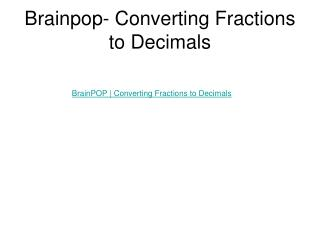DownloadDownload PresentationBrainpop- Converting Fractions to Decimals

# Brainpop- Converting Fractions to Decimals

Télécharger la présentation## Brainpop- Converting Fractions to Decimals

- - - - - - - - - - - - - - - - - - - - - - - - - - - E N D - - - - - - - - - - - - - - - - - - - - - - - - - - -
##### Presentation Transcript

1. Brainpop- Converting Fractions to Decimals BrainPOP | Converting Fractions to Decimals

2. Fractions Decimals Decimal Fraction The place value tells you the denominator of the fraction. Then simplify your answer Fraction Decimal To write a fraction as a decimal, the denom. needs to be a power of 10.Find a power of 10 that the denom. will fit into evenly. Then multiply both the numerator and demon. by that factor. 0.4 The tenths place 2 5 4 10 X 2 X 2 0.4 4 10 = = = You can also divide the numerator by the denominator to change a fraction to a decimal. Add to your notes http://www.amblesideprimary.com/ambleweb/mentalmaths/fracto.html

3. Calculator Decimal Fraction Type in your decimal: 0.6 Remember to hit until you no longer see the symbol Fraction Decimal Type in your fraction: F D 2 5 F D = Simp = n d Correct answer is 0.4 Correct answer is 3/5 Add to your notes